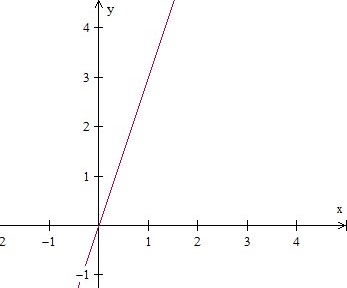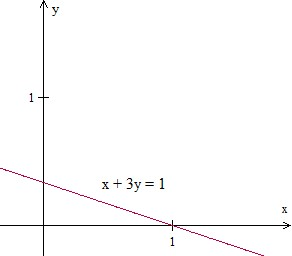# Graphing a line in quadrant 1

#### Excel Pivot Tables and Dashboard. Simple & Quick tutorial!

Most Popular

7 Lectures 58 mins

#### Learn HTML Tables

15 Lectures 59 mins

#### Excel Analytics - Data Analysis with Pivot-Tables and Charts

46 Lectures 2.5 hours

We graph a line whose equation is given, say for example y = 3x. We need at least two points or ordered pairs to graph the line. First we choose some x values. Then we evaluate y = 3x for each value of x.

For example for x = 0, y = 3(0) = 0; x = 1, y = 3(1) = 3 and so on. We put the x, y and the ordered pair (x, y) values as follows.

x y (x,y)
0 3(0) = 0 (0,0)
1 3(1) = 3 (1,3)
2 3(2) = 6 (2,6)
3 3(3) = 9 (3,9)
4 3(4) = 12 (4,12)

We see that the ordered pairs lie in the quadrant 1. Joining the points will give the graph of the line in quadrant 1.Graph the line in the quadrant 1, whose equation is given below.

2x – y = 3

### Solution

Step 1:

Given equation 2x – y = 3; for y = 0, x = 3/2; for x = 2, y = 2(2)–3 = 4–3 = 1. So two ordered pairs are (3/2, 0) (2, 1)

Step 2:

Plotting the points and joining with a line we getGraph the line in the quadrant 1, whose equation is given below.

x + 3y = 1

### Solution

Step 1:

Given equation x + 3y = 1; for y = 0, x = 1; For x = 0, y = 1/3. So two ordered pairs are (1, 0) and (0, 1/3)

Step 2:

Plotting the points and joining with a line we get